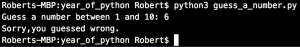## Day 2: A real classic – guess a number with Python

Today we code a little app that will take in a users number between 1 and 10. The program will generate a random number between 1 and 10 and will compare the two. If the user guessed right, he will get a friendly message and vice versa.The Code:

``````from random import randint #so we can use randint

def guess_number(your_guess):
random_nr = randint(1,10) # generate a random number between 1 and 10
if (int(your_guess)==random_nr):
print("You guessed right!")
else:
print("Sorry,you guessed wrong.")
return

guess_number(input("Guess a number between 1 and 10: "))``````

/